# Part 3: Algebraic Techniques | Free Worksheet

Many questions in Mathematics require you to have fluency with Algebraic Techniques.. We've put this article together to help you master this topic in Year 7. Read on for explanations, worked examples and checklist questions with solutions!Algebra is a central part of most Maths topics in High School. You’ve got to get it right early on. But don’t worry in this article, you’re going to learn all about Year 7 algebraic techniques!

## NSW Syllabus Outcome

 NESA Syllabus Outcomes Syllabus Outcome Explanation Recognise like terms and add and subtract them to simplify algebraic expressions This means that you will be able to answer questions like $$5a + 7a + 3b$$ and $$8a + 3b – 2b$$ Simplify algebraic expressions that involve multiplication and division This means that you can simplify $$18b \div 2b$$ Expand algebraic expressions by removing grouping symbols This means that you know how to expand $$3(x+4)$$

We will be covering the following topics:

And you can check your knowledge and skills on some:

## Assumed knowledge

This guide lays the foundation for Algebraic Techniques.

To succeed in this topic, students should be comfortable computing with integers and fractions and have familiarity with basic index notation.

## Test your knowledge of Algebra before we get started!## Simplifying Expressions

Pronumerals essentially represent numbers, and, hence, they can be added, subtracted, multiplied and divided – the four number operations.

Let’s look at this in more detail.

### Addition and Subtraction – Collecting like terms

Lengthy expressions such as $$3a + 2a + a + b + 2b + 3b$$ can be written in a much shorter form. This is known as simplifying the expression.

The key aspect of this process is that only like terms can be combined. Hence, the process is called “collecting like terms”.

The below examples illustrate this process of simplification.

Examples

Simplify the following:

1. $$3a + 2a + a + b + 2b + 3b$$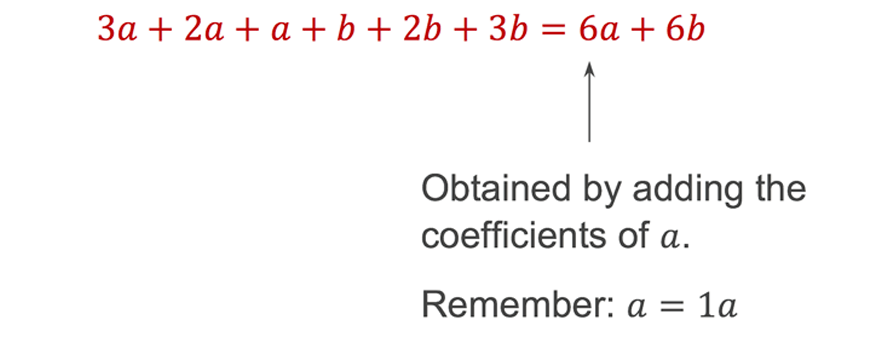2. $$4ab – 2ba$$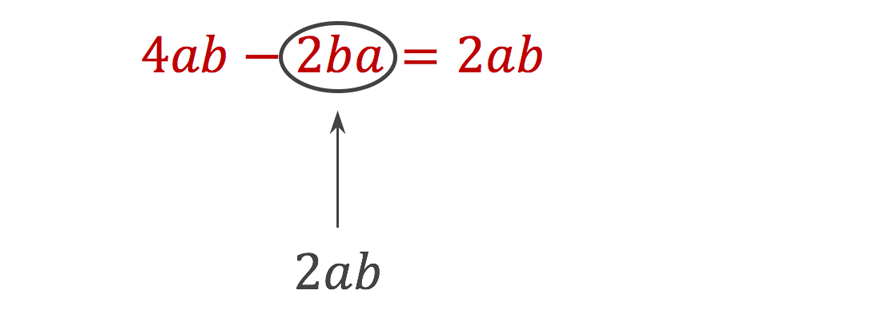3. $$-5x^{2} + 3x – 2x^{2} + x$$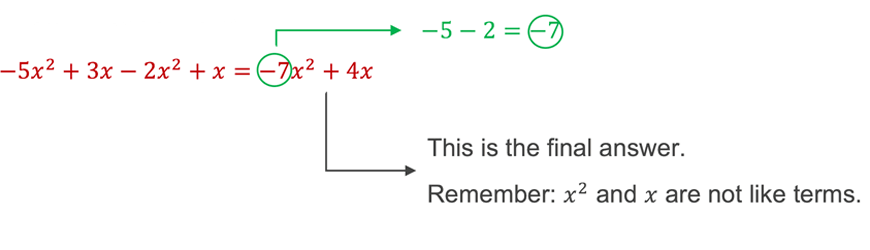Common Mistakes

Expressions with unlike terms cannot be simplified further.

A common mistake is writing $$2x + 3y$$ as $$5xy$$.
i.e. writing $$2x + 3y = 5xy$$.

$$2x$$  and $$3y$$ are unlike terms. So, they must be kept as separate terms when added.

A good way to remember avoiding mistake is to note that $$x + y$$ will not produce $$xy$$.

Similar variants of the above mistake include:

• $$x + 5x^{2} = 6x^{3}$$
• $$x + 3 = 3x$$

### Multiplication and Division

Unlike addition and subtraction, like terms are not necessary for multiplication and division.

The rule for this process is to operate the numbers with the numbers, and the letters with the letters.

Let’s have a look at this in action.

Examples

Simplify the following:

1. $$7a \times 9b$$

\begin{align*}
\color{red}{7a \times 9b = 63ab}
\end{align*}

2. $$8u \times u$$

\begin{align*}
\color{red}{8u \times u = 8u^{2}}
\end{align*}

3. $$(5t)^{3}$$

\begin{align*}
\color{red}{(5t)^3 = 5t \times 5t \times 5t = 125t^{3}}
\end{align*}

Division can be written as a fraction. Fractions can be simplified by cancelling.

Remember that cancelling means to divide two terms by a common factor and that you can only cancel vertically or diagonally.

Let’s have a look at cancelling in action.

Examples

Simplify the following

1. $$18b \div 3$$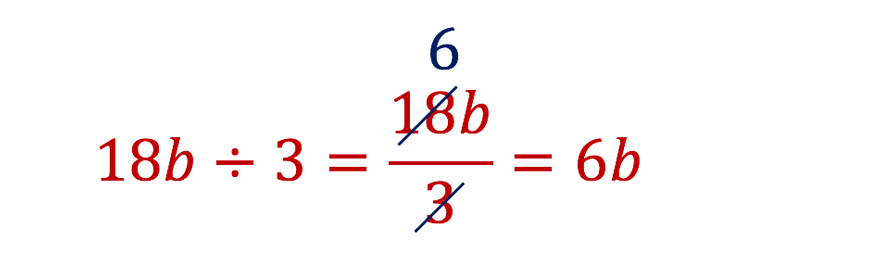2. $$15xy^{2}t \div 5xy$$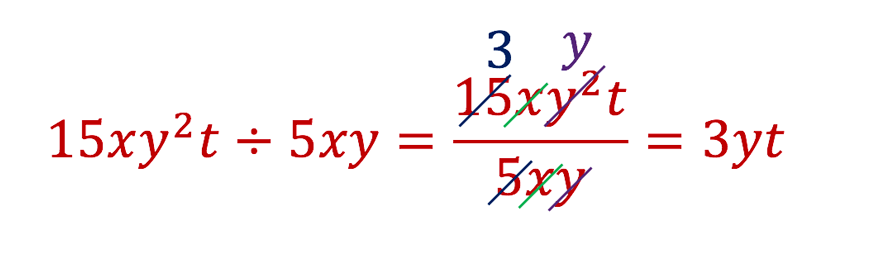3. $$25x^{7}y^{4} \div 5x^{3}y^{5}$$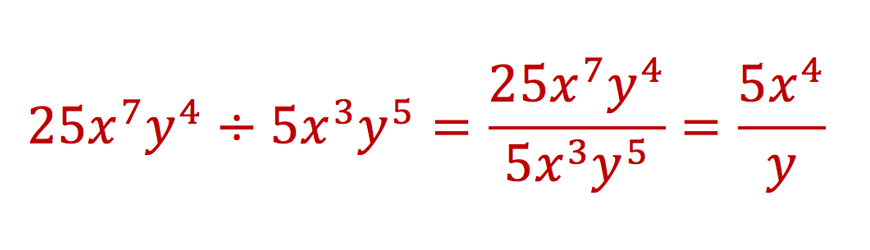4. $$\frac{2x^{3}y^{5}}{5z^{10}} \div \frac{6x^5y^2}{z}$$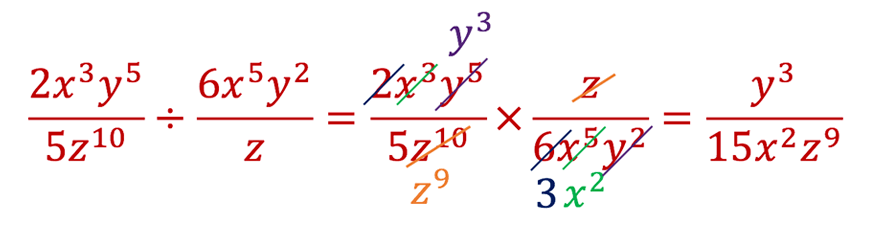## The Distributive Law

Simplifying expressions should always be performed as it allows for questions to be handled more easily.

However, it is sometimes necessary to expand an expression, such as $$2(3x + 5y) + 5(x – 2y)$$ before like terms can be collected.

Expanding expressions involves using the distributive law: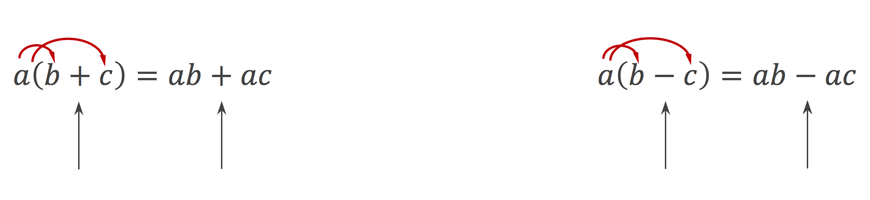Let’s see this at work.

Examples

Expand the following:

1. $$5(a + 3)$$

\begin{align*}
\color{red}{5(a + 3) = 5a + 15}
\end{align*}

2. $$b(3b -a)$$

\begin{align*}
\color{red}{b(3b -a) = 3b^{2} – ab}
\end{align*}

3. $$-3(x-2)$$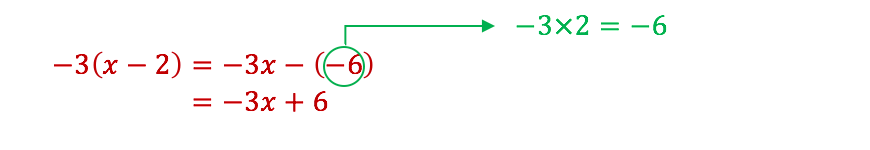A faster way is to do:This method is used for the rest of this subject Guide.Note: $$-3 \times -2 = 6$$

4. $$-(x-5)$$

\begin{align*}
\color{red}{-(x-5) = -x + 5}
\end{align*}

Expand and simplify the following:

1. $$3(x+4) + 2(x-3)$$

\begin{align*}
\color{red}{3(x+4) + 2(3-3)} &\color{red}{= 3x + 12 + 2x – 6} \\
&\color{red}{= 5x+6}
\end{align*}

2. $$4(2a-3) – 3(2-a)$$

\begin{align*}
\color{red}{4(2a-3) – 3(2-a)} & \color{red}{= 8a -12 -6 + 3a} \\
&\color{red}{= 11a – 18}
\end{align*}

3. $$3y(2x – 3) + \frac{2}{3}(3x-y)$$

\begin{align*}
\color{red}{3y(2x – 3) + \frac{2}{3}(3x-y)} & \color{red}{= 2xy + 3y + \frac{2}{3}x – \frac{2}{3}y }\\
&\color{red}{= 2xy + \frac{2}{3}x + \frac{7}{3}y}
\end{align*}

Now we’ve covered the skills, it’s time for you to put them into action!

## Checkpoint Questions

1. Simplify $$6a + 4a – 3a$$

2. Simplify $$m^{2} + m + 3m^{2} + 4{m}$$

3. Simplify $$-3a \times 2b$$

4. Simplify $$4 \times -b \times -c$$

5. Simplify $$12x^{2}y \div 4xy$$

6. Simplify $$2x^{4}y \div 3x^{3}y^{2}$$

7. Expand $$-21(2-3a)$$

8. Expand and simplify $$5p(2p-1) – 3(p-2)$$

9. Simplify $$\frac{(2x^{3})^{2}y^{5}}{5z^{5}} \div \frac {5x^{5}y^{2}}{3z} \div 2x$$

10. Simplify $$\frac{\frac{3}{2}x – \big{(}\frac{1}{3}x-2\big{)} + \frac{2}{5}x}{3 \big{(}\frac{2}{5}x-2\big{)} + 4\big{(}\frac{11}{120}x+2\big{)}}$$

## Build your child’s algebraic skills now!## Solution

1.

\begin{align*}
6a + 4a – 3a = 7a
\end{align*}

2.

\begin{align*}
m^{2} + m + 3m^{2} + 4m = 4m^{2} + 5m
\end{align*}

3.

\begin{align*}
-3a \times 2b = -6ab
\end{align*}

4.

\begin{align*}
4 \times -b \times -c
\end{align*}

5.

\begin{align*}
12x^{2y} \div 4xy = \frac{12x^{2}y}{4xy} = 3x
\end{align*}

6.

\begin{align*}
2x^{4}y \div 3x^{3}y^{2} = \frac{2x^{4}y}{3x^{3}y^{2}} = \frac{2x}{3xy}
\end{align*}

7.

\begin{align*}
-2a(2-3a) = -4a+6a^{2}
\end{align*}

8.

\begin{align*}
5p(2p-1) – 3(p-2) &= 10p^{2} – 5p -3p + 6 \\
&= 10p^{2} – 8p + 6
\end{align*}

9. Note that $$(2x^{3})^{2} = 2x^{3} \times 2x^{3} = 4x^{6}$$

\begin{align*}
∴ \frac{(2x^{3})^{2}y^{5}}{5z^{5}} \div \frac{5x^{5}y^{2}}{3z} \div 2x &= \frac{4x^{6}y^{5}}{5z^{5}} \times \frac{3z}{5x^{5}y^{2}} \times \frac{1}{2x} \\
&= \frac{6y^3}{25z^{4}}
\end{align*}

10.

\begin{align*}
\frac{\frac{3}{2}x \big{(}\frac{1}{3}x – 2 \big{)} + \frac{2}{5}x}{3\big{(}\frac{2}{5}x-2\big{)} + 4 \big{(} \frac{11}{120} + 2 \big{)}} & =\frac{\frac{3}{2}x – \frac{1}{3}x + 2 + \frac{2}{5}x}{\frac{6}{5}x – 6 + \frac{11}{30}x + 8} \\
&= \frac{\frac{45}{30}x – \frac{10}{30}x + 2 + \frac{12}{30}x}{\frac{36}{30}x – 6 + \frac{11}{30}x + 8} \\
&= \frac{\frac{47}{30}x + 2}{\frac{47}{30}x + 2} \\
&= 1
\end{align*}

© Matrix Education and www.matrix.edu.au, 2020. Unauthorised use and/or duplication of this material without express and written permission from this site’s author and/or owner is strictly prohibited. Excerpts and links may be used, provided that full and clear credit is given to Matrix Education and www.matrix.edu.au with appropriate and specific direction to the original content.